Like   Tweet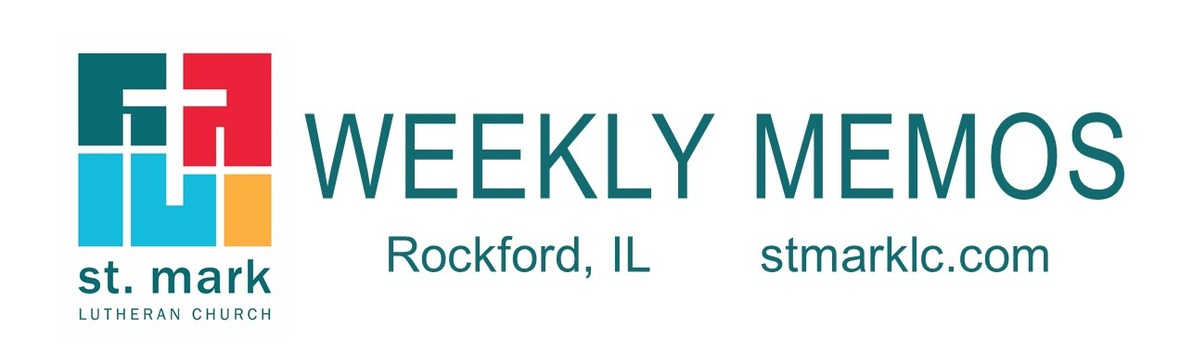table div table+table div table{width:100%;padding:0}table div table+table div table img{width:96.23%;padding:0;float:none}table div table+table div table td{width:100%;padding:0 1.88% 18px}/* styles */## Pastoral Reflection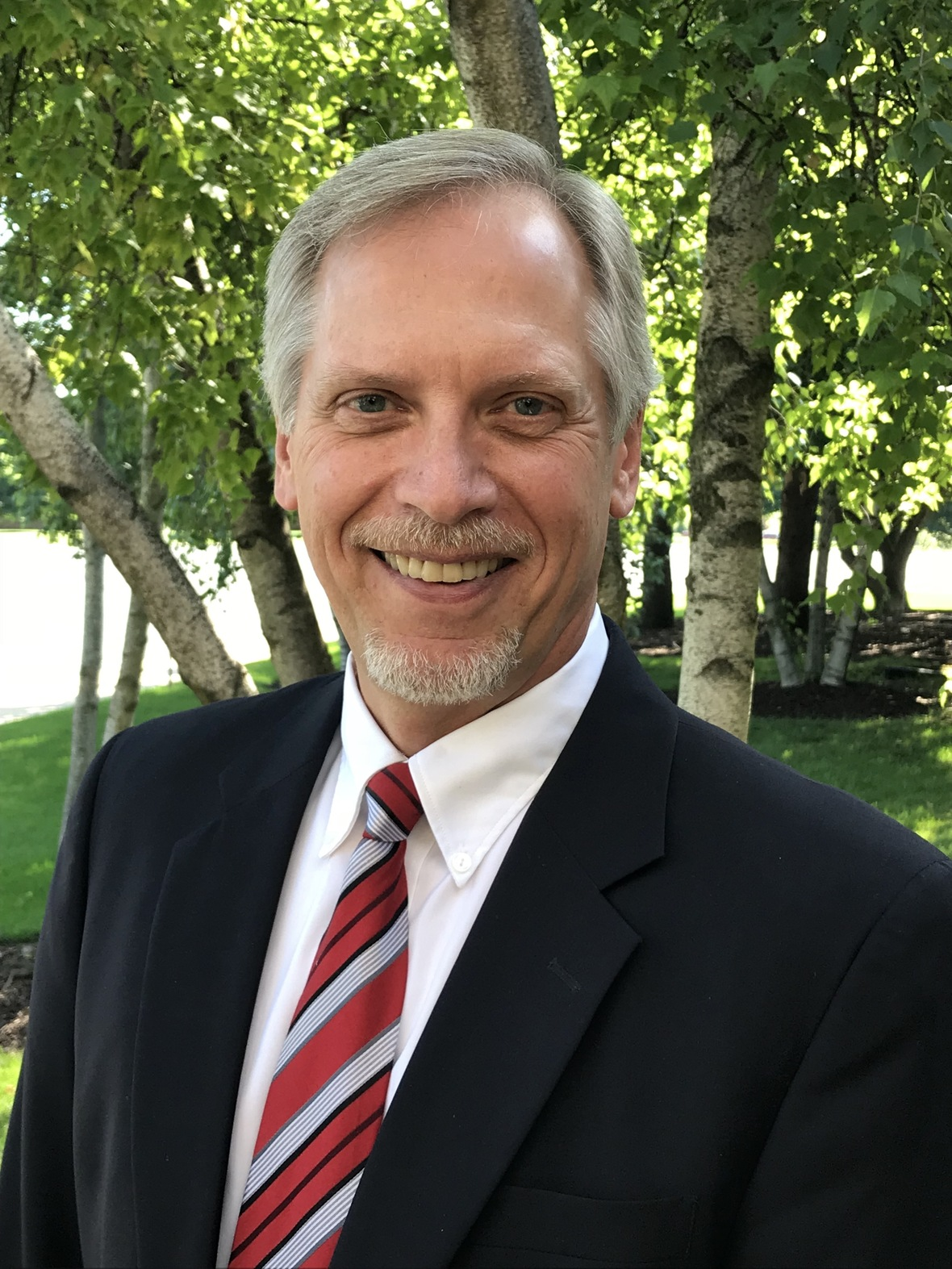With Gratitude
Pastor Mark Hagen

I began my sermon last Sunday acknowledging that my retirement was close at hand. Read more.

 table div table+table+table+table+table div table{width:100%;padding:0}table div table+table+table+table+table div table img{width:96.23%;padding:0;float:none}table div table+table+table+table+table div table td{width:100%;padding:0 1.88% 18px}/* styles */## News and Events

 table div table+table+table+table+table+table+table div table{width:100%;padding:0}table div table+table+table+table+table+table+table div table img{width:96.23%;padding:0;float:none}table div table+table+table+table+table+table+table div table td{width:100%;padding:0 1.88% 18px}/* styles */Since we will not be together on Pastor Mark’s official last Sunday, May 31, we are throwing him a “Drive Through Retirement Parade.”

We want the entire congregation to come out and support Pastor Mark, thank him for his faithful ministry of 15 years to St. Mark, and wish him well on his retirement. Honk your car horns for the man who has made a difference in so many of our lives. We are asking for you to come to St. Mark on Sunday, May 31 between 1:00 and 3:00 P.M., rain or shine. If you have a card for Pastor Mark, please bring it to the parade, we will have a basket for you to put it in.

Remember this is a parade and that means that you don’t get out of your car to hug Pastor Mark (even if you want to) or shake his hand. He will be outside under the new canopy waiting for you to driveby. You can decorate your vehicle with a message to him on poster board, add streamers, balloons, and even bring a noisemaker or two. You will want to decorate the drivers side of your vehicle since this will be the side that Pastor Mark will be seeing. So get in your vintage car, convertible, or motorcycle and join the parade.

We ask for you to enter St. Mark from Featherstone Road (the entrance east of the building by the playground), and exit through the Medical Office Building parking lot on the south side. The Mulford entrance will be blocked off. There will be people directory you once you enter the parking lot around the building to Pastor. Mark. Please see the map below.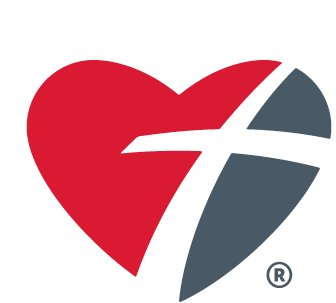CARE Packages for the Caregivers
During our parade, we will be collecting snack items that will be put into care packages for the caregivers/staff at area nursing homes. Individual packs of cookies, chips, granola bars, and candy are all encouraged. The care package will also include a copy of Pastor Mark's book. This collection is happening with help from an action grant from Thrivent.

As you drive around the church, there will be a location that we will have helpers, wearing masks, collecting the snack items from your car.

 table div table+table+table+table+table+table+table+table+table+table div table{width:100%;padding:0}table div table+table+table+table+table+table+table+table+table+table div table img{width:96.23%;padding:0;float:none}table div table+table+table+table+table+table+table+table+table+table div table td{width:100%;padding:0 1.88% 18px}/* styles */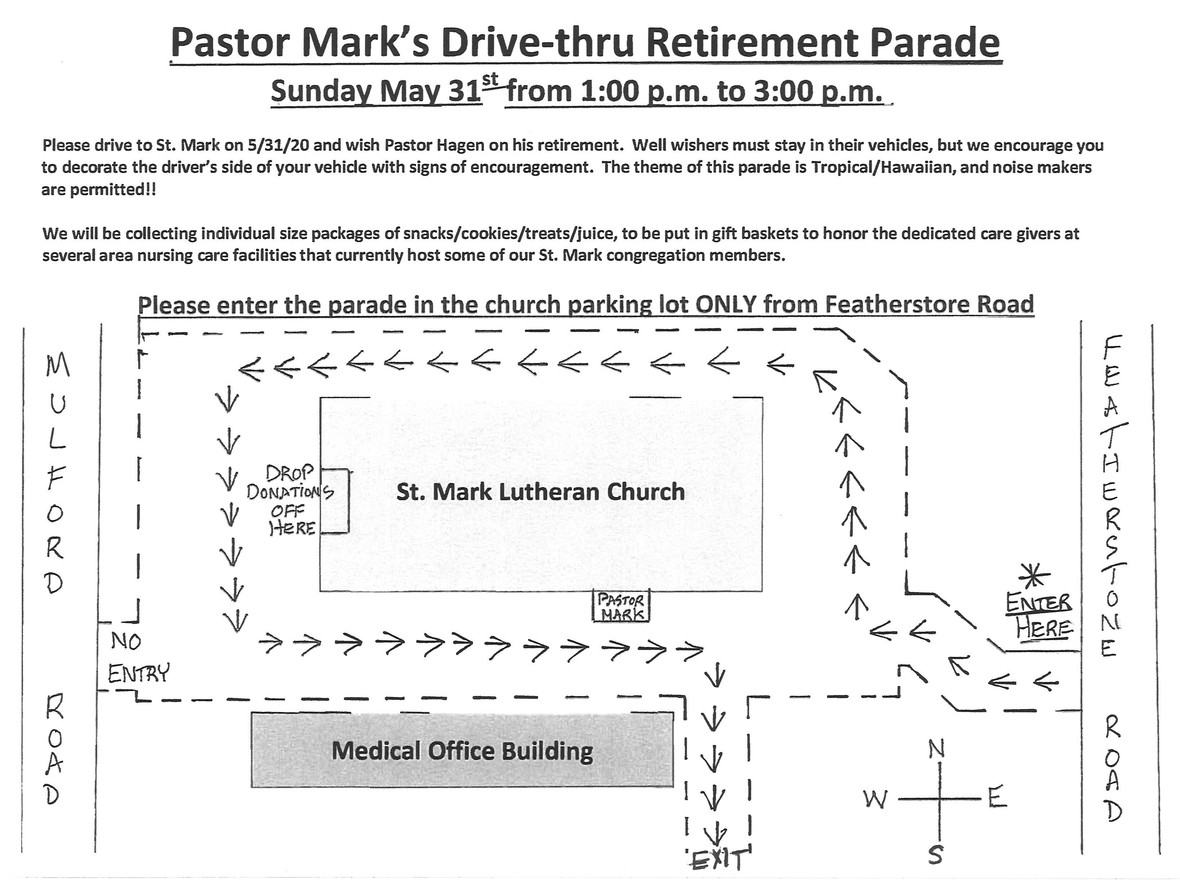table div table+table+table+table+table+table+table+table+table+table+table+table div table{width:100%;padding:0}table div table+table+table+table+table+table+table+table+table+table+table+table div table img{width:96.23%;padding:0;float:none}table div table+table+table+table+table+table+table+table+table+table+table+table div table td{width:100%;padding:0 1.88% 18px}/* styles */Pastor Mark's Book Signing
Many of you have asked if you could have your book signed by Pastor Mark and the answer is yes. The plan is to bring the book that you have purchased to the parade, Sunday, May 31 from 1:00 - 3:00 P.M. If the book is yours, please write your first and last name inside the book and place it in a sealed Ziploc bag. If you have purchased the book to give to someone, write your name and who the book is for on a post-it note and place the book in a sealed Ziploc bag. We will have a location at the parade for you to hand this to someone. Pastor Mark will take these books and sign all of them. The books will be back in the office for you to come by and pick up from 10 - Noon on Wednesday, June 3, Friday, June 5, and Monday, June 8. Please only come on these days as we will have someone at the church during those times.

 table div table+table+table+table+table+table+table+table+table+table+table+table+table+table div table{width:100%;padding:0}table div table+table+table+table+table+table+table+table+table+table+table+table+table+table div table img{width:96.23%;padding:0;float:none}table div table+table+table+table+table+table+table+table+table+table+table+table+table+table div table td{width:100%;padding:0 1.88% 18px}/* styles */Dear friends,
I am delighted to announce the completion of my book, Tears and Triumph: A Journey Toward Resurrection. I began this writing project nine months ago while on sabbatical, and I am eager to finally share my story. I am grateful for the encouragement and support you’ve given me during this precious time of reflection and writing. I pray that you will likewise discover encouragement and hope in the reading of it. This book is available in both Kindle and Paperback editions, only through Amazon. Please use this link to order your copy. Pastor Mark Hagen

 table div table+table+table+table+table+table+table+table+table+table+table+table+table+table+table+table div table{width:100%;padding:0}table div table+table+table+table+table+table+table+table+table+table+table+table+table+table+table+table div table img{width:96.23%;padding:0;float:none}table div table+table+table+table+table+table+table+table+table+table+table+table+table+table+table+table div table td{width:100%;padding:0 1.88% 18px}/* styles */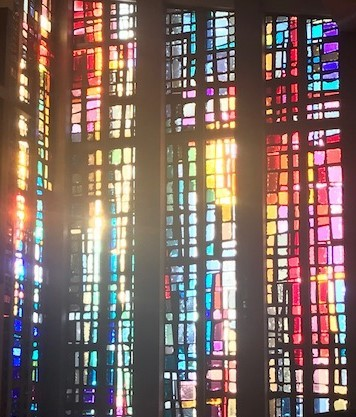You can enjoy Sunday's worship anywhere you have the internet. On your phone, laptop, iPad, or if you have a smart tv. So grab a cup of coffee or tea, and join our St. Mark family in worship.

We will be streaming abbreviated, pre-recorded worship services on our St. Mark YouTube Channel, available for viewing throughout the day on Sundays, beginning at 8:00 A.M.

Look for a special email and push notification from our app on Sunday morning providing a link to this service on YouTube. We hope this is bringing a much-needed sense of community during these difficult times of separation.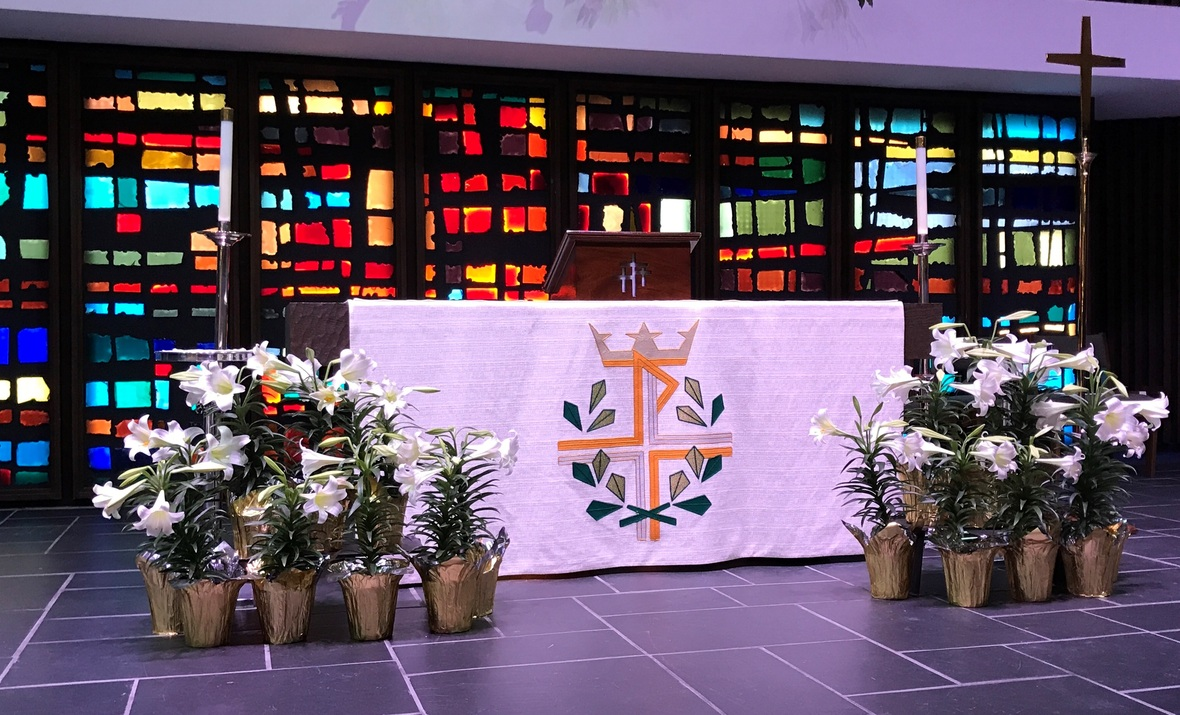table div table+table+table+table+table+table+table+table+table+table+table+table+table+table+table+table+table+table+table div table{width:100%;padding:0}table div table+table+table+table+table+table+table+table+table+table+table+table+table+table+table+table+table+table+table div table img{width:96.23%;padding:0;float:none}table div table+table+table+table+table+table+table+table+table+table+table+table+table+table+table+table+table+table+table div table td{width:100%;padding:0 1.88% 18px}/* styles */St. Mark Mobile App!

This is different than our church directory app.
Our mobile app is designed specifically for St. Mark to improve our communication with our congregation and nurture our members. We encourage you to download the app. It's about getting involved and staying connected.

 table div table+table+table+table+table+table+table+table+table+table+table+table+table+table+table+table+table+table+table+table+table div table{width:100%;padding:0}table div table+table+table+table+table+table+table+table+table+table+table+table+table+table+table+table+table+table+table+table+table div table img{width:96.23%;padding:0;float:none}table div table+table+table+table+table+table+table+table+table+table+table+table+table+table+table+table+table+table+table+table+table div table td{width:100%;padding:0 1.88% 18px}/* styles */Congratulations!
Matthew Boucher, Jacob Crowell, Jackson Graber, Zach Hamann, Emma Harring, Courtney Hinrichs, Anna Hornick, Adam Kunar, Olivia Kuss, Cole Panjkovich, Josh Sattler, Aidan Westerberg, Olivia Zaluckyj.

 table div table+table+table+table+table+table+table+table+table+table+table+table+table+table+table+table+table+table+table+table+table+table+table div table{width:100%;padding:0}table div table+table+table+table+table+table+table+table+table+table+table+table+table+table+table+table+table+table+table+table+table+table+table div table img{width:96.23%;padding:0;float:none}table div table+table+table+table+table+table+table+table+table+table+table+table+table+table+table+table+table+table+table+table+table+table+table div table td{width:100%;padding:0 1.88% 18px}/* styles */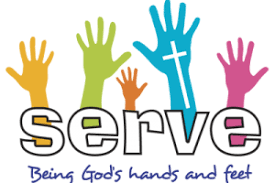Summer Mission Trip has had to be re-imagined!
We are not traveling, but we are happening!
Our mission organizer Service Learning Camp has encouraged us that service/mission is more needed than ever. But we are no longer going to Ohio.

The “Summer of Service” is being planned for local mission work in Rockford July 12-15th. This event is for youth entering high school fall 2020-12th grade. We will have a home base at St.Mark and doing service out in the community. The cost is just \$50! The fee may be paid on our app or if you don't have the mobile app, click here to pay on Pushpay.

Sign-up by June 12. Please text or call Melinda Alekna 815-871-0390.

 table div table+table+table+table+table+table+table+table+table+table+table+table+table+table+table+table+table+table+table+table+table+table+table+table+table div table{width:100%;padding:0}table div table+table+table+table+table+table+table+table+table+table+table+table+table+table+table+table+table+table+table+table+table+table+table+table+table div table img{width:96.23%;padding:0;float:none}table div table+table+table+table+table+table+table+table+table+table+table+table+table+table+table+table+table+table+table+table+table+table+table+table+table div table td{width:100%;padding:0 1.88% 18px}/* styles */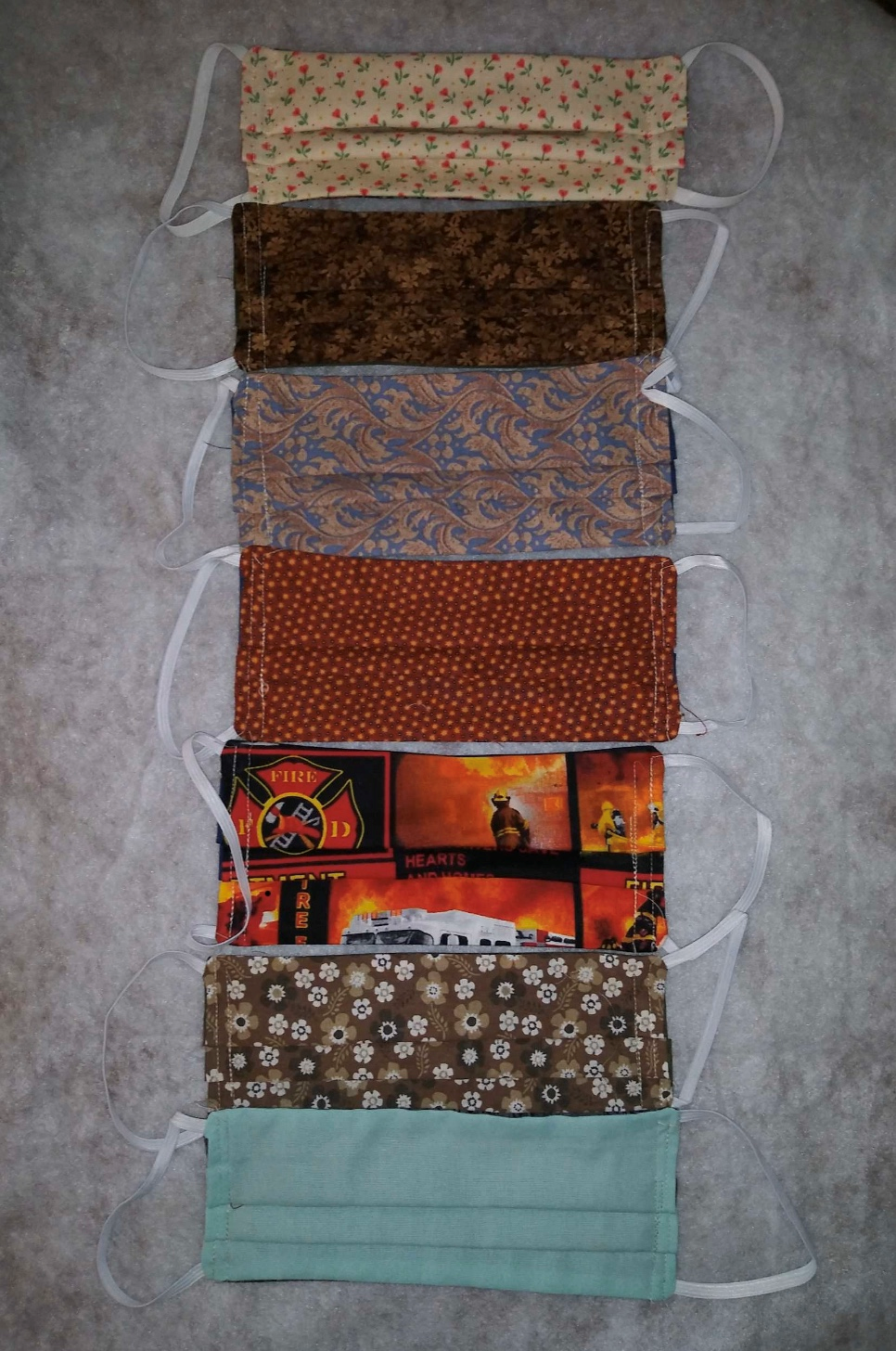Thank you to the Sew and Sews who have been incredibly busy. They are each making 100’s of face masks. The just finished making over 200 “port pillows” for Chemo Cargo. St. Mark is truly blessed by our Sew and Sews!

Because of their generosity, their heart, and skills, the sewers are making masks for the congregation, as needed. There will be a bin of masks, outside the south doors. Monday, Wednesday, and Friday from 10:00 A.M. till Noon. If you need masks dropped to your residence, children size, or questions text/call Melinda Alekna 815-871-0390. If you would like to help with delivering to people, we could use your help as well.

 table div table+table+table+table+table+table+table+table+table+table+table+table+table+table+table+table+table+table+table+table+table+table+table+table+table+table+table div table{width:100%;padding:0}table div table+table+table+table+table+table+table+table+table+table+table+table+table+table+table+table+table+table+table+table+table+table+table+table+table+table+table div table img{width:96.23%;padding:0;float:none}table div table+table+table+table+table+table+table+table+table+table+table+table+table+table+table+table+table+table+table+table+table+table+table+table+table+table+table div table td{width:100%;padding:0 1.88% 18px}/* styles */table div table+table+table+table+table+table+table+table+table+table+table+table+table+table+table+table+table+table+table+table+table+table+table+table+table+table+table+table+table div table{width:100%;padding:0}table div table+table+table+table+table+table+table+table+table+table+table+table+table+table+table+table+table+table+table+table+table+table+table+table+table+table+table+table+table div table img{width:96.23%;padding:0;float:none}table div table+table+table+table+table+table+table+table+table+table+table+table+table+table+table+table+table+table+table+table+table+table+table+table+table+table+table+table+table div table td{width:100%;padding:0 1.88% 18px}/* styles */I want to thank you for your continued support of St. Mark through your financial gifts to our ministry. During this difficult time, we have fallen behind in our offerings and need your assistance. I would kindly ask that you revisit your pledge for this year and strive to fulfill your weekly giving. While our ministry feels like it’s “on hold,” our financial commitments continue...and we need your help to stay current. Again, I extend my appreciation for your faithfulness and support.

Together, we will get through this time more resilient and focused as a congregation. I am grateful for your witness to the power of God’s Spirit to lead us! ~ Pastor Mark

 table div table+table+table+table+table+table+table+table+table+table+table+table+table+table+table+table+table+table+table+table+table+table+table+table+table+table+table+table+table+table+table div table{width:100%;padding:0}table div table+table+table+table+table+table+table+table+table+table+table+table+table+table+table+table+table+table+table+table+table+table+table+table+table+table+table+table+table+table+table div table img{width:96.23%;padding:0;float:none}table div table+table+table+table+table+table+table+table+table+table+table+table+table+table+table+table+table+table+table+table+table+table+table+table+table+table+table+table+table+table+table div table td{width:100%;padding:0 1.88% 18px}/* styles */Vacation Bible School Update
In response to the Covid-19 pandemic, and in anticipation of extended restrictions on large gatherings to help control the spread of the illness, VBS will not be held the week of June 15. Instead, it will be postponed until late summer. Planning and preparation will continue in the hope of a joyful return to each other’s company in the coming months!

 table div table+table+table+table+table+table+table+table+table+table+table+table+table+table+table+table+table+table+table+table+table+table+table+table+table+table+table+table+table+table+table+table+table div table{width:100%;padding:0}table div table+table+table+table+table+table+table+table+table+table+table+table+table+table+table+table+table+table+table+table+table+table+table+table+table+table+table+table+table+table+table+table+table div table img{width:96.23%;padding:0;float:none}table div table+table+table+table+table+table+table+table+table+table+table+table+table+table+table+table+table+table+table+table+table+table+table+table+table+table+table+table+table+table+table+table+table div table td{width:100%;padding:0 1.88% 18px}/* styles */With the state's "Stay At Home" executive order in place, the St. Mark office will be closed and staff will be working from home. Should you need assistance from the office staff, you may email jill@stmarklc.com and someone will be in touch.

We do have a staff person going to church every day to check the mailbox, voicemails and what ever needs to be attended to.

We ask for your patience and prayers as we face this pandemic together. While we can’t predict what’s immediately ahead of us, we can live our days with assurance and faith in the Lord of the Church to be with us.

 table div table+table+table+table+table+table+table+table+table+table+table+table+table+table+table+table+table+table+table+table+table+table+table+table+table+table+table+table+table+table+table+table+table+table+table div table{width:100%;padding:0}table div table+table+table+table+table+table+table+table+table+table+table+table+table+table+table+table+table+table+table+table+table+table+table+table+table+table+table+table+table+table+table+table+table+table+table div table img{width:96.23%;padding:0;float:none}table div table+table+table+table+table+table+table+table+table+table+table+table+table+table+table+table+table+table+table+table+table+table+table+table+table+table+table+table+table+table+table+table+table+table+table div table td{width:100%;padding:0 1.88% 18px}/* styles */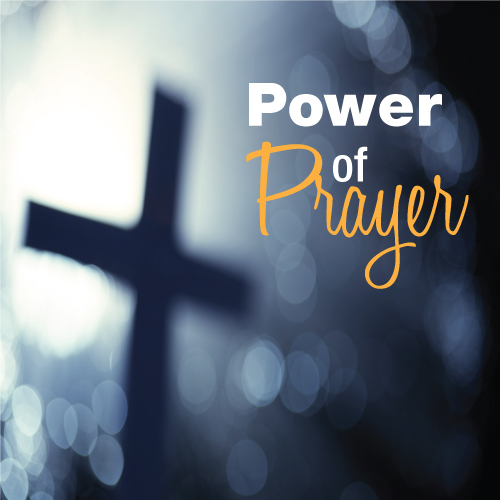St. Mark Prayer Chain
Would you like to be a part of the St. Mark prayer chain? Prayer requests come into the church office weekly. As we receive prayer requests we would send out an e-mail and ask that you pray daily for the persons mentioned in the requests. All requests are to remain confidential. Please e-mail melinda@stmarklc.com if you would like to participate in the prayer chain. “Be anxious for nothing, but in everything by prayer and supplication, with thanksgiving, let your requests be made known to God.” Colossians 4:2.

 table div table+table+table+table+table+table+table+table+table+table+table+table+table+table+table+table+table+table+table+table+table+table+table+table+table+table+table+table+table+table+table+table+table+table+table+table+table div table{width:100%;padding:0}table div table+table+table+table+table+table+table+table+table+table+table+table+table+table+table+table+table+table+table+table+table+table+table+table+table+table+table+table+table+table+table+table+table+table+table+table+table div table img{width:96.23%;padding:0;float:none}table div table+table+table+table+table+table+table+table+table+table+table+table+table+table+table+table+table+table+table+table+table+table+table+table+table+table+table+table+table+table+table+table+table+table+table+table+table div table td{width:100%;padding:0 1.88% 18px}/* styles */Pastor Mark's Retirement Celebration Dinner Postponed
The committee has been meeting via Zoom trying their best to plan a very special celebration for Pastor Mark, but due to the recent conditions, we are under they have decided to postpone.

At this time we are not sure how the months ahead will look at how large gatherings will happen. We are very excited about planning his much-needed celebration, but we will hold off on a date as of now.

Be patient, and have hope and strength for better days ahead. We will keep you posted on what the plans will be.

 table div table+table+table+table+table+table+table+table+table+table+table+table+table+table+table+table+table+table+table+table+table+table+table+table+table+table+table+table+table+table+table+table+table+table+table+table+table+table+table div table{width:100%;padding:0}table div table+table+table+table+table+table+table+table+table+table+table+table+table+table+table+table+table+table+table+table+table+table+table+table+table+table+table+table+table+table+table+table+table+table+table+table+table+table+table div table img{width:96.23%;padding:0;float:none}table div table+table+table+table+table+table+table+table+table+table+table+table+table+table+table+table+table+table+table+table+table+table+table+table+table+table+table+table+table+table+table+table+table+table+table+table+table+table+table div table td{width:100%;padding:0 1.88% 18px}/* styles */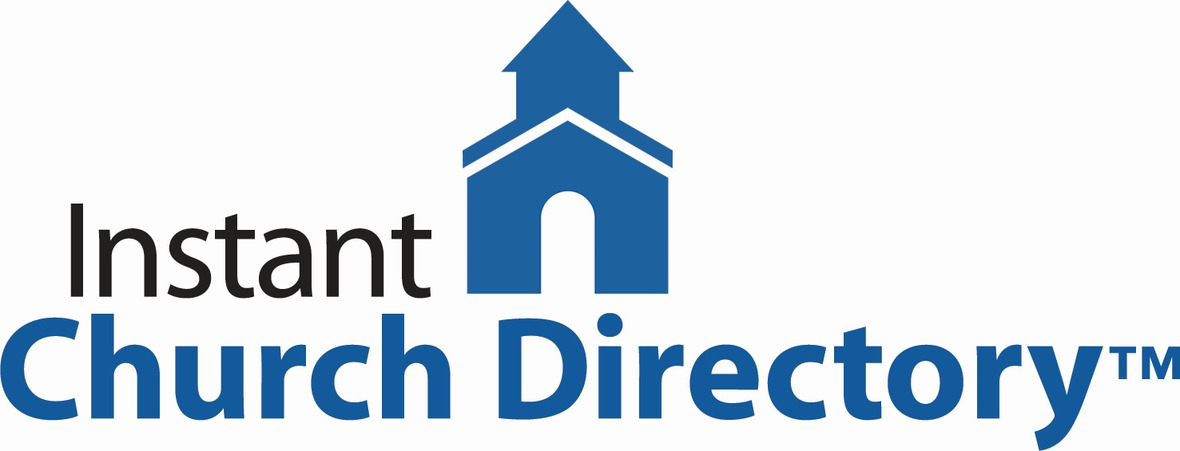Instant Church Directory
This is a great time to stay connected with our St. Mark family. If you need help downloading the app or creating an account on your computer or ipad, please call Jill Davenport at 815-218-3403 or email jill@stmarklc.com and she will be happy to help you through the process.

It is simple and takes only a few minutes to upload your photo and download or create an online account on your computer or kindle. The Instant Church Directory App. You will always have updated members' information at your fingertips.

Instant Church Directory

Mobile App Login Instructions for Android & Kindle Devices

We also have a form for you to complete to make sure we have the most current information for the directory. There are paper forms at church or click on the link to Church Directory Form

 table div table+table+table+table+table+table+table+table+table+table+table+table+table+table+table+table+table+table+table+table+table+table+table+table+table+table+table+table+table+table+table+table+table+table+table+table+table+table+table+table+table div table{width:100%;padding:0}table div table+table+table+table+table+table+table+table+table+table+table+table+table+table+table+table+table+table+table+table+table+table+table+table+table+table+table+table+table+table+table+table+table+table+table+table+table+table+table+table+table div table img{width:96.23%;padding:0;float:none}table div table+table+table+table+table+table+table+table+table+table+table+table+table+table+table+table+table+table+table+table+table+table+table+table+table+table+table+table+table+table+table+table+table+table+table+table+table+table+table+table+table div table td{width:100%;padding:0 1.88% 18px}/* styles */## Outreach Ministry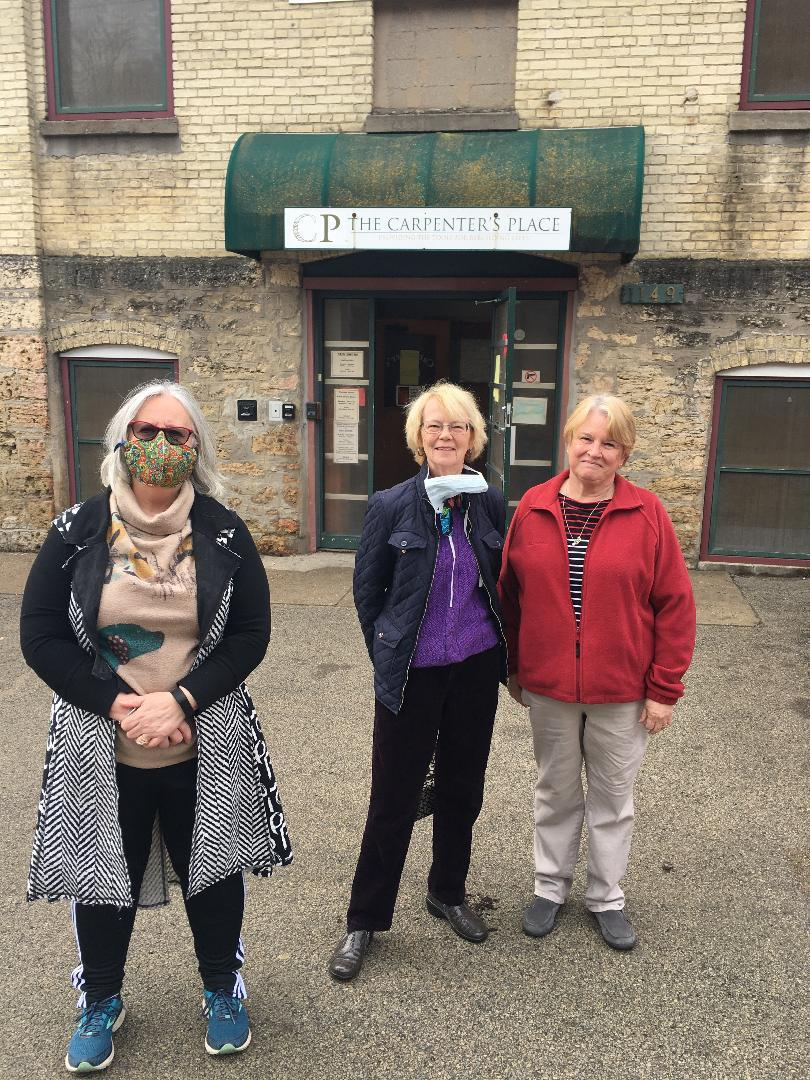/* styles */ Outreach thanks the following brave St. Mark Volunteers who prepared and brought tuna noodle casserole, cookies, and milk to Carpenters Place on April 23. We thank Kim Semmelroth, Claire Brown, Sue Peterson, and Diane Starbuck (not pictured). St. Mark Volunteers provide a noon lunch at Carpenters Place every other month.
 table div table+table+table+table+table+table+table+table+table+table+table+table+table+table+table+table+table+table+table+table+table+table+table+table+table+table+table+table+table+table+table+table+table+table+table+table+table+table+table+table+table+table+table+table+table div table{width:100%;padding:0}table div table+table+table+table+table+table+table+table+table+table+table+table+table+table+table+table+table+table+table+table+table+table+table+table+table+table+table+table+table+table+table+table+table+table+table+table+table+table+table+table+table+table+table+table+table div table img{width:96.23%;padding:0;float:none}table div table+table+table+table+table+table+table+table+table+table+table+table+table+table+table+table+table+table+table+table+table+table+table+table+table+table+table+table+table+table+table+table+table+table+table+table+table+table+table+table+table+table+table+table+table div table td{width:100%;padding:0 1.88% 18px}/* styles */## Barnabas Ministry

 /* styles */ Dear Friend – I love my early morning quiet time with the Lord. I’m in a really interesting Bible, called The Cultural Background Bible – giving me the background of each passage like never before. On Friday morning I came to Acts 4 – the foundational text for our Barnabas Caregiving together. 32-33 The whole congregation of believers was united as one—one heart, one mind! They didn’t even claim ownership of their own possessions. No one said, “That’s mine; you can’t have it.” They shared everything. The apostles gave powerful witness to the resurrection of the Master Jesus, and grace was on all of them. 34-35 And so it turned out that not a person among them was needy. Those who owned fields or houses sold them and brought the price of the sale to the apostles and made an offering of it. The apostles then distributed it according to each person’s need. 36-37 Joseph, called by the apostles “Barnabas” (which means “Son of Comfort”), a Levite born in Cyprus, sold a field that he owned, brought the money, and made an offering of it to the apostles. Our one mission is to encourage – by listening... Our homebound loved ones in institutions have never been more alone, because of Covid-19 restrictions. They need our encouragement more than ever. For now, pray without ceasing for everyone Melinda names in our prayer chain – daily, at length. AND – plan to come to our Barnabas meetings when we can resume. Dr. Paul Tillich says, The first duty of love is to listen. How faithful. How Barnabas! May the Lord watch between me and thee – Pastoral Care Pastor Chuck Olson
 table div table+table+table+table+table+table+table+table+table+table+table+table+table+table+table+table+table+table+table+table+table+table+table+table+table+table+table+table+table+table+table+table+table+table+table+table+table+table+table+table+table+table+table+table+table+table+table+table div table{width:100%;padding:0}table div table+table+table+table+table+table+table+table+table+table+table+table+table+table+table+table+table+table+table+table+table+table+table+table+table+table+table+table+table+table+table+table+table+table+table+table+table+table+table+table+table+table+table+table+table+table+table+table div table img{width:96.23%;padding:0;float:none}table div table+table+table+table+table+table+table+table+table+table+table+table+table+table+table+table+table+table+table+table+table+table+table+table+table+table+table+table+table+table+table+table+table+table+table+table+table+table+table+table+table+table+table+table+table+table+table+table div table td{width:100%;padding:0 1.88% 18px}/* styles */table div table+table+table+table+table+table+table+table+table+table+table+table+table+table+table+table+table+table+table+table+table+table+table+table+table+table+table+table+table+table+table+table+table+table+table+table+table+table+table+table+table+table+table+table+table+table+table+table+table+table div table{width:100%;padding:0}table div table+table+table+table+table+table+table+table+table+table+table+table+table+table+table+table+table+table+table+table+table+table+table+table+table+table+table+table+table+table+table+table+table+table+table+table+table+table+table+table+table+table+table+table+table+table+table+table+table+table div table img{width:96.23%;padding:0;float:none}table div table+table+table+table+table+table+table+table+table+table+table+table+table+table+table+table+table+table+table+table+table+table+table+table+table+table+table+table+table+table+table+table+table+table+table+table+table+table+table+table+table+table+table+table+table+table+table+table+table+table div table td{width:100%;padding:0 1.88% 18px}/* styles */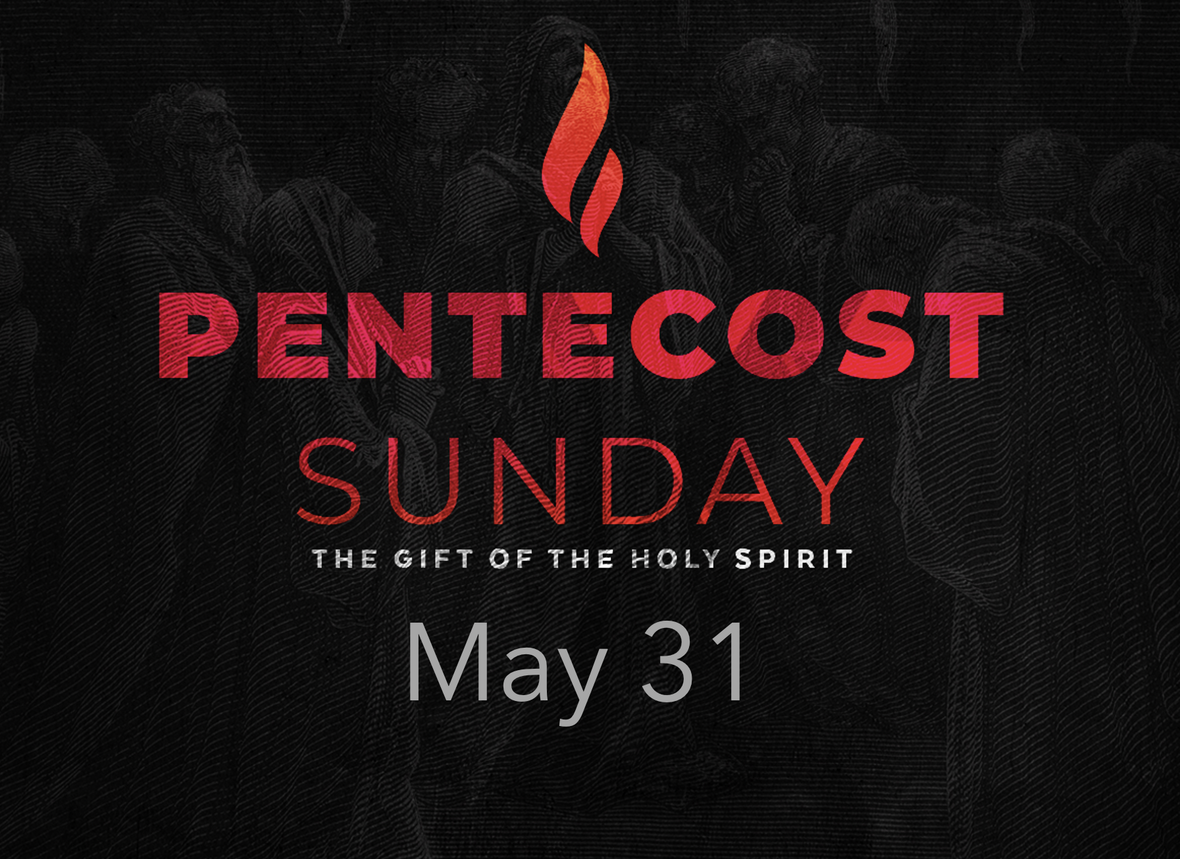table div table+table+table+table+table+table+table+table+table+table+table+table+table+table+table+table+table+table+table+table+table+table+table+table+table+table+table+table+table+table+table+table+table+table+table+table+table+table+table+table+table+table+table+table+table+table+table+table+table+table+table+table div table{width:100%;padding:0}table div table+table+table+table+table+table+table+table+table+table+table+table+table+table+table+table+table+table+table+table+table+table+table+table+table+table+table+table+table+table+table+table+table+table+table+table+table+table+table+table+table+table+table+table+table+table+table+table+table+table+table+table div table img{width:96.23%;padding:0;float:none}table div table+table+table+table+table+table+table+table+table+table+table+table+table+table+table+table+table+table+table+table+table+table+table+table+table+table+table+table+table+table+table+table+table+table+table+table+table+table+table+table+table+table+table+table+table+table+table+table+table+table+table+table div table td{width:100%;padding:0 1.88% 18px}/* styles */## Our St. Mark members:

Joe Fink, Dorothy Brommerich, Gary Campbell, Bill Jennings, Tim Panjkovich, Bette Patterson, Jason Carlson, Beverly Wright, Jerry Cazaniga, David Young, Keith Haggestad.

## Family and friends of St. Mark members:

the Ferguson family, Gayle Coffler, Darold, Beekmann, Janet Webster, Rae Ann McIntire, Mark Cotter, Lynda Foxley, Shirley Drummond, Linda Johnson, Nancy Kunnert, Robert and Cindy Wilson, Pastor Jane McChesney, Emily Tropp, Louis Fivaz, Mike Leonard, Katelynn Audette, Eva Love Sherbondy, Clay Heinrich, Mary Graham, Kim Menke, Norma Comstock, Carolyn King, Ashton Henley, Nathan Gyllin.

## Those serving in the military and their families:

Hunter Haggestad, Tristan Davenport, Scot Hornick, Bailey Panjkovich, Xander Gehrke, Jim Tammen, Brandon Ballenger, Taylor Eversole, Dean Barron.

Strengthen and encourage those in public health services and in the medical profession: care-givers, nurses, attendants, doctors, all who commit themselves to care for the sick and their families.

 table div table+table+table+table+table+table+table+table+table+table+table+table+table+table+table+table+table+table+table+table+table+table+table+table+table+table+table+table+table+table+table+table+table+table+table+table+table+table+table+table+table+table+table+table+table+table+table+table+table+table+table+table+table+table+table div table{width:100%;padding:0}table div table+table+table+table+table+table+table+table+table+table+table+table+table+table+table+table+table+table+table+table+table+table+table+table+table+table+table+table+table+table+table+table+table+table+table+table+table+table+table+table+table+table+table+table+table+table+table+table+table+table+table+table+table+table+table div table img{width:96.23%;padding:0;float:none}table div table+table+table+table+table+table+table+table+table+table+table+table+table+table+table+table+table+table+table+table+table+table+table+table+table+table+table+table+table+table+table+table+table+table+table+table+table+table+table+table+table+table+table+table+table+table+table+table+table+table+table+table+table+table+table div table td{width:100%;padding:0 1.88% 18px}/* styles *//* styles */ Office Hours Currently, the staff is working from home. You can contact them through email. The church directory app and our St. Mark app has the staff contact information, or you can leave a message on the church's voicemail and someone will get back to you as soon as possible. Telephone: 815-398-3557 Publications St. Mark Memos, the weekly e-newsletter, is distributed each Thursday. The deadline for submission is Monday by 4:00 P.M. Please click to send submissions. Sermons Online Miss a Sunday at St. Mark? Please visit our website and watch the sermons online. Please click to watch.